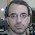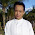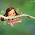## Tuesday, January 22, 2013

### Electronic Circuit DC Motor Driver using H-Bridge MOSFET IRF9540 and IRF540

Robometricschool. - One again that we can design DC motor driver using H-bridge MOSFET. In here we find term about H-Bridge. H-Bridge is a form of hardware network that serves to move the motor.  It is called H-Bridge because the schematic like a bridge that can arrange from MOSFET IRF9540 and IRF540.

Electronic Circuit of DC Motor Driver using MOSFET IRF9540 and IRF540Figure 1. DC Motor Driver using H-Bridge MOSFET IRF9540 and IRF540

Circuit Performance

According electronic schematic DC Motor Driver using MOSFET IRF9540 and IRF540 like in figure 1 above we know that this schematic using H-Bridge that arrange from two IRF9540 and two IRF540 with input using optocoupler PC123. To know configuration of PC123 you can see figure 2 bellow:Figure 2. PC123 configuration

IRF9540 is MOSFET channel P and IRF540 is MOSFET channel N that be used to control motor to rotate or not rotate. We can control motor rotate with follow:
1. Input A = 0 and Input B = 0, motor will not rotate because all MOSFET IRF9540 work and All MOSFET IRF540 not work.
2. Input A = 1 and Input B = 0, motor will rotate anti clock wise because all MOSFET IRF9540 Q2 and MOSFET IRF540 Q3 work, other MOSFET not work.
3. Input A = 0 and Input B = 1, motor will rotate clock wise because all MOSFET IRF9540 Q1 and MOSFET IRF540 Q4 work, other MOSFET not work.
4. Input A = 1 and Input B = 1, motor will not rotate because all MOSFET IRF540 work and All MOSFET IRF9540 not work.
Note:
• With this circuit schematic in figure 1, we get good security although we give same logical in input A and input B.
• We can get more power with change all MOSFET series according with current that allowed in MOSFET datasheet.

1.Hi, Thank you very much for the schema , it is very helpful.

I must say tath there is a conexion mising , from the motor to the junction of drains of the Mosfets, by making tath conexion , I've tested with motors and it works perfect , and it supports a lot of current ,

Gero

2.Thanks for mentioning it, I felt that something was not right. I am trying to design an h bridge to use as amplifier on a rfid system. Would you tell me which values of resistors did you use, as well as diodes? How much current you reckon it is capable to drain?

Héctor

1.please use R3 and R4 with 1K ohm, R1 and R4 with 4K7/1watts

3.This comment has been removed by the author.

4.Hi,
Here, what are ratings of resiator and diode???

5.What is the rating of diode used in this circuit. What is the output current from these circuit

6.What is the rating of diode used in this circuit. What is the output current from these circuit

7.In the diagram it was already told about an error. The engine (a point 1) needs to be connected to a joint wire of Q1-Q3, "a point 2" to a joint wire of Q2-Q4. Q1-Q4 transistors have the built-in protective diodes, on it D1-D4 diodes in the diagram are not necessary.